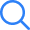# NCERT Solutions For Class 7 MathsNCERT Solutions Class 7 Maths Chapter wise with Exercise Wise helps students to solve all the questions given in the Class 7 NCERT Mathematics Textbooks. In order to understand the seven standard mathematics, students are required to solve all the questions. Therefore, the NCERT Solutions for Class 7 maths PDF is available here for free of cost.

These can be downloaded in PDF file format and can be used while doing homework or final exam preparation. The chapter-wise and Exercise wise links to download the solutions are given in the below sections.

## NCERT Solutions Class 7 Maths Chapter Wise PDFTo be able to answer all the questions asked in the examination, it is imperative for the students to be well versed with the complete chapter of Maths. Practicing the chapter wise questions enables the students to know a bunch of different topics in detail. Along with solving the problems, it helps in covering the syllabus completely that can result in good marks.

While solving the questions students are needed to answer them completely by referring to all the essential formulas and studied concepts. For that reason the Class 7 NCERT Solutions that are prepared by keeping in mind the students' understanding level can be helpful. The chapter-wise NCERT Solutions of class 7th are given in the table. Students can download these solutions in English medium here. For now,  NCERT solutions for class 7 Maths in Hindi Medium isn't available on Selfstudys but, we will try our best to provide them here as soon as possible.

Once it will be available you will be able to access NCERT Solutions for class 7 maths in Hindi medium PDF. But for now, download English medium Class 7th mathematics solutions from the below given table.

 NCERT Solutions for Class 7 Maths Chapter Wise Chapter 1 Integers Chapter 2 Fractions and Decimals Chapter 3 Data Handling Chapter 4 Simple Equations Chapter 5 Lines and Angles Chapter 6 The Triangles and its Properties Chapter 7 Congruence of Triangles Chapter 8 Comparing Quantities Chapter 9 Rational Numbers Chapter 10 Practical Geometry Chapter 11 Perimeter and Area Chapter 12 Algebraic Expressions Chapter 13 Exponents and Powers Chapter 14 Symmetry Chapter 15 Visualising Solid Shapes

### NCERT Solutions for Class 7 Maths Chapter 1 IntegersClass 7 NCERT Maths Chapter 1 Integers covers basics but one of the most important topics such as sums on integer operations, commutative property, distributive property, associativity, and additive identity, etc.

This chapter allows students to learn and see the importance of integers from different perspectives. With 4 exercises along with 30 questions, it helps students to understand the whole concept thoroughly. Links to download exercise wise answers are given in the table. Feel free to use them.

#### NCERT Solutions Class 7 Maths Chapter 1 Integers (Exercise:- 1.1 - 1.4)### NCERT Solutions for Class 7 Maths Chapter 2 Fractions and DecimalsClass 7 NCERT Maths Chapter 2 Fractions and Decimals is not some new topic. Those students who are studying in class VII are already aware of this, since they have learned it in earlier classes. However, in this standard, this topic has been broadened a bit.

Good knowledge of this chapter is essential because in further classes more questions will be introduced on this topic. Although in class 7 Fractions and Decimals chapter helps students to learn Multiplication and division of fractions along with simplifying fractions to their lowest form, etc.

In a total of 7 exercises consisting of 48 questions, this chapter gives a good understanding of Fractions and Decimals.

#### NCERT Solutions Class 7 Maths Chapter 2 Fractions and Decimals (Exercise:- 2.1 - 2.7)### NCERT Solutions for Class 7 Maths Chapter 3 Data HandlingBefore performing the computations with the help of data, it is important to organize them. Thus, the Class 7 NCERT Maths Chapter 3 Data Handling helps the learners to know how to collect, organize data, represent them, use bar graphs with a different purposes, etc.

There are a total of 4 exercises that comprises a total of 24 questions. Out of 24 questions approx 4 questions are a bit tough. However, no need to worry because the given exercise-wise solutions will help in answering them with ease.

#### NCERT Solutions Class 7 Maths Chapter 3 Data Handling(Exercise:- 3.1 - 3.4)### NCERT Solutions for Class 7 Maths Chapter 4 Simple EquationsChapter 4 Simple Equations allow the learners to understand how to convert a statement into an equation by taking the help of addition and subtraction. In this chapter, you will get to know how to transpose numbers in the equations. It also allows students to learn the implications of simple equations in real-life situations. It has a total of 4 exercises which have 18 questions.

#### NCERT Solutions Class 7 Maths Chapter 4 Simple Equations (Exercise:- 4.1 - 4.4)### NCERT Solutions for Class 7 Maths Chapter 5 Lines and AnglesClass 7 NCERT Maths Chapter 5 Lines and Angles gives students a good insight into lines, angels and their properties. This chapter covers concepts of parallel lines, intersection of lines and the pairs of angles, linear pair, vertically opposite angles, etc. A total of 20 questions available throughout the 2 exercises allow students to have a good sense of this chapter. Links to download those 2 exercises solutions are given in the table here. It is free to access in PDF file format.

#### NCERT Solutions Class 7 Maths Chapter 5 Lines and Angles (Exercise:- 5.1 - 5.2)### NCERT Solutions for Class 7 Maths Chapter 6 The Triangle and Its PropertiesChapter 6 The Triangle and Its Properties has a total of 5 exercises and 21 questions. This chapter gives students in-depth knowledge of triangles and its properties. This chapter covers the concepts of the median of a triangle, altitude of a triangle, exterior angle of a triangle, etc. The Pythagoras property is also explained in it. The learners can refer to this chapter's solutions to practice the given questions and to understand the topics more precisely. Links are available in the given table here.

#### NCERT Solutions Class 7 Maths Chapter 6 The Triangle and Its Properties (Exercise:- 6.1 - 6.5)### NCERT Solutions for Class 7 Maths Chapter 7 Congruence of TrianglesClass 7 NCERT Chapter 7 Congruence of Triangles is an extended version of the triangle chapter where the topics of congruence have been discussed extensively. When two triangles have the same shape and size they are said to be congruent. Therefore, this chapter aids students to know more about them in-depth.

With the help of 2 exercises and 14 questions, different criteria such as SSS, SAS, ASA, and RHS have been used to teach students the congruence of triangles.

#### NCERT Solutions Class 7 Maths Chapter 7 Congruence of Triangles (Exercise:- 7.1 - 7.2)### NCERT Solutions for Class 7 Maths Chapter 8 Comparing QuantitiesClass 7 NCERT Maths Chapter 8 Comparing Quantities is one of the most important topics since the topics taught in this lesson are being used often in the real world. It gives students the ability to convert fractional numbers into percentages, the ratio in percentage, profit and loss into percentages, Simple Interest, etc.

Students can find 3 exercises and a total of 24 questions. Where each question is of different difficulty levels, from easy to a bit challenging. The download links of class 7 solutions are given in the table.

#### NCERT Solutions Class 7 Maths Chapter 8 Comparing Quantities (Exercise:- 8.1 - 8.3)### NCERT Solutions for Class 7 Maths Chapter 9 Rational NumbersChapter 9 Rational Numbers gives the opportunity to learn the representation of rational numbers on the number line, and standard form, along with the operations on rational numbers. There are a total of 2 exercises that contain a total of 14 questions from easy to difficult.

A good practice of the given chapter allows the students to make a strong base so that in later classes they can use this topic effortlessly. Download Free PDF Class 7 Maths Chapter 9 from the links present in the table here.

#### NCERT Solutions Class 7 Maths Chapter 9 Rational Numbers (Exercise:- 9.1 - 9.2)### NCERT Solutions for Class 7 Maths Chapter 10 Practical GeometryPractical Geometry Chapter 10 of NCERT Class 7 enables the learners to learn how to construct the transversals and parallel lines with the help of geometrical instruments. Constructing triangles using SSS, SAS, ASA, and RHS criteria are also part of this chapter. So, this chapter needs rigorous practice in order to understand it faster and easier.

However, there are already 5 exercises in this chapter that have a total of 16 questions. In order to get access to solutions to those questions refers to the given table here.

#### NCERT Solutions Class 7 Maths Chapter 10 Practical Geometry (Exercise:- 10.1 - 10.5)## NCERT Solutions for Class 7 Maths Chapter 11 Perimeter and AreaThis chapter is very straightforward and simple. Perimeter and Area is a part of mensuration where students get to know about Determining the area and perimeter of closed figures such as squares, rectangles, triangles, parallelograms, and circles. With 4 exercises and 44 problems, it gives good practice to this chapter. In order to get hints while you are stuck on some questions, you can refer to the exercise-wise solutions given in the table. It is free of cost.

#### NCERT Solutions Class 7 Maths Chapter 11 Perimeter and Area (Exercise:- 11.1 - 11.4)### NCERT Solutions for Class 7 Maths Chapter 12 Algebraic Equations7th Class Maths Chapter 12 Algebraic Equations help the students learn how to form simple mathematical statements into algebraic equations, find the value of an expression, etc. It covers plenty of topics such as addition, and subtraction of like terms, types of polynomials, etc. There are 4 exercises and 25 curated problems on the given topics.

Taking help of the given Solutions can aid in solving all types of questions whether it is tough or easy.

#### NCERT Solutions Class 7 Maths Chapter 12 Algebraic Equations (Exercise:- 12.1 - 12.4)### NCERT Solutions for Class 7 Maths Chapter 13 Exponents and PowersExponents and Powers assist students to learn how to convert an exponent into a number and vice versa. In addition to that, it allows students to learn about the laws of exponents and apply them to problems. All the problems have been given in 3 different exercises. Solving those problems helps in understanding the use of Exponents and Powers more rigorously.

#### NCERT Solutions Class 7 Maths Chapter 13 Exponents and Powers (Exercise:- 13.1 - 13.3)### NCERT Solutions for Class 7 Maths Chapter 14 SymmetryChapter 14 Symmetry for regular polygons, rotational symmetry, line symmetry and rotational symmetry are covered in this chapter. Symmetry means similar. So, the given exercise in this lesson will allow the students to learn about the similarity of shapes. It also gives a general idea of symmetrical use in the real world. In Class 7th chapter 14 there are 3 exercises. Practice them thoroughly to get a good grasp on them.

#### NCERT Solutions Class 7 Maths Chapter 14 Symmetry (Exercise:- 14.1 - 14.3)### NCERT Solutions for Class 7 Maths Chapter 15 Visualising Solid ShapesClass 7 NCERT Maths Chapter 15 Visualising Solid Shapes teaches students how to recognize the different elements of a solid figure. This chapter deals with plane figures and solids, faces, edges, vertices, 3D Shapes, etc. The concepts of this chapter have been explained throughout 4 exercises. Extensive practice of it can give a good control on shapes. Furthermore, referring to the solutions can ease in answering the problems more easily. Download links are given here in the table.

#### NCERT Solutions Class 7 Maths Chapter 15 Visualising Solid Shapes (Exercise:- 15.1 - 15.4)### Chapter Wise Study Tips For Class 7 Maths

All the students studying in CBSE Class 7 have one aim: to get full marks in NCERT Class 7 Maths.

There are lots of study methods to get full marks in class 7 maths but, here we have mentioned the chapter wise study tips that will aid students like you to study and prepare for maths to score higher marks.

#### Integers (Chapter 1)

NCERT Class 7th Maths Chapter 1 Integers contains a total of 4 exercises in which 30 questions are asked. The properties of arithmetic operations are the main focus of this lesson. The Properties of Addition, Subtraction, division and multiplication of Integers are covered.

In other words, the chapter Integers took the learning of whole numbers and integers a bit further. As per the definition the integers are called a bigger collection of whole numbers and negative numbers. However, these topics have been already covered in class 6 but in class 7 this topic has been broadened into various sections.

1.1 Introduction:- This section contains the basic definition of the integer and what was covered in the previous class.

1.2 Recall:- It deals with the representation of integers on a number line which has been already taught in class 6. However, with the purpose of revision, in the recall section various examples have been given.

1.3 Properties Of Addition And Subtraction Of Integers:- This section has been divided into 5 subsections: (1.3.1) Closure under addition, (1.3.2) closure under subtraction, (1.3.3) commutative property, (1.3.4) associative property, (1.3.5)additive identity

1.4 Multiplication Of Integers:- After teaching students how to add and subtract integers, section 1.4 explains how to multiply integers. Furthermore, this section has been broken into 3 different subsections: Multiplication of a positive and a negative integer, multiplication of two negative integers, product of three or more negative integers.

1.5 Properties Of Multiplication Of Integers:- After covering the method to multiply the integers, the section 1.5 covers the properties of multiplication of integers in 7 subsections: (1.5.1) Closure under multiplication, (1.5.2) Commutativity of multiplication, (1.5.3) multiplication by zero, (1.5.4) multiplicative identity, (1.5.5) Associativity for multiplication, (1.5.6) distributive property, (1.5.7) Making Multiplication Easier.

1.6 Division Of Integers:- From the basic knowledge of division, we know that the inverse operation of multiplication is known as the division. So, this section 1.6 of chapter 1 integers, covers how to perform division with the integers.
1.7 Properties Of Division Of Integers:- After teaching how to divide integers, the section 1.7 explains about the properties of division of integers such as division is not commutative for integers, any integer a, a ÷ 0 is not defined but, 0 ÷ a = 0 for a ≠ 0.

Integers Chapter 1 Exercises

Ex. 1.1 – 10 Questions

Ex. 1.2 – 4 Questions

Ex. 1.3 – 9 Questions

Ex. 1.4 – 7 Questions

Important Formula of Class 7 Integers

1. Commutative Property: a + b = b + a; a × b = b × a
2. Associative Property: (a + b) + c = a + (b + c); (a × b) × c = a × (b × c)
3. Distributive Property: a × (b + c) = a × b + a × c
4. Multiplicative Identity: 1 × a = a × 1 = a
5. Additive Identity: a + 0 = 0 + a = a

Study Tips for Class 7 Integers

The basic understanding of integers’ definition, their important formulas and lots of practice to the questions are essential study tips for class 7 Integers.

However, a student’s main focus should be on developing the core knowledge of this topic.

#### Fractions and Decimals (Chapter 2)

NCERT gives the definition of Fraction as a part of a group. In other words, A fraction is a numerical representation for part of a whole.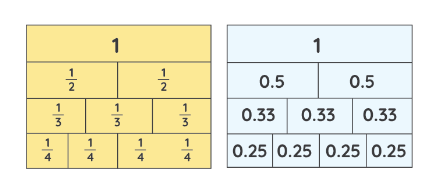So, this chapter deals with the various categories of fractions and how to work with them.

Furthermore, it lets students understand how to multiply and divide the fractions.

As students proceed further in the chapter they get to know the decimals and how to perform multiplication and division by using the fraction and decimals.

This topic has been explained in detail throughout the 7 subsections:

2.1 Introduction:- The introduction part of Chapter 2 gives students a brief of what topics in fractions and decimals they have studied in the previous class. Along with that equivalent fractions, representation of fractions on the number line and ordering of fractions have been introduced in class 6 but, here in standard 7th fractions and decimals have been given a different heights, where learners will learn multiplication and division of fractions as well as of decimals.

2.2 How Well Have You Learnt About Fractions?:- Since the fraction has been introduced in class 6th , the NCERT textbook of class 7th has asked the students in section 2.2 about how well they have learnt about fractions. In this section, it has been explained with the examples. Proper fraction, Improper fraction, & mixed fractions are elaborated with the ideal examples and by giving the definitions.

2.3 Multiplication Of Fractions:- By relating to the previously studied topics of finding the area, the section 2.3 helps students to know how to multiply fractions. By giving the example of What will be the area of the rectangle if its length and breadth are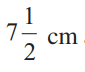and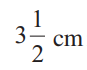respectively? The textbooks tried to explain this in the easiest manner.

Apart from this, there are two subsections: (2.3.1) Multiplication of a Fraction by a Whole Number & (2.3.2 ) Multiplication of a Fraction by a Fraction.

2.4 Division Of Fractions:- The section 2.4 division of fractions have been also elaborated in two subsections: (2.4.2) Division of a Fraction by a Whole Number, (2.4.3) Division of a Fraction by Another Fraction

2.5 How Well Have You Learnt About Decimal Numbers:- Section 2.5 aims to help students recall their basic learning of decimals. Here is the table for the recall purpose.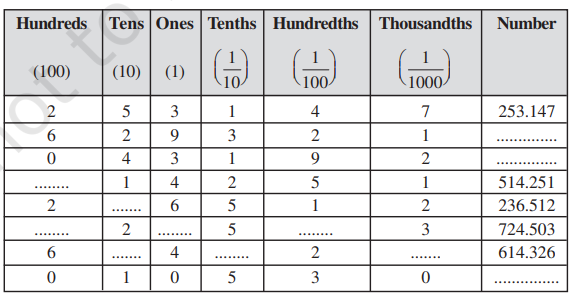2.6 Multiplication Of Decimal Numbers:- The knowledge of decimals has been further broadened in section 2.6 by introducing the Multiplication of decimal numbers.

In the subsection (2.6.1 )Multiplication of Decimal Numbers by 10, 100 and 1000, the level of this topic has been given a new height.

2.7 Division Of Decimal Numbers:- There are three subsections: (2.7.1) Division by 10, 100 and 1000, (2.7.2 ) Division of a Decimal Number by a Whole Number, (2.7.3 ) Division of a Decimal Number by another Decimal Number. In all of these subsections the division of decimal numbers has been explained.

Fractions and Decimals Chapter 2 Exercises

Ex. 2.1 – 8 Questions

Ex. 2.2 – 8 Questions

Ex. 2.3 – 8 Questions

Ex. 2.4 – 4 Questions

Ex. 2.5 – 5 Questions

Ex. 2.6 – 9 Questions

Ex. 2.7 – 6 Questions

Study Tips for Class 7 Fractions and Decimals

The chapter 2 covers the basics level topics hence it is important to read all of them thoroughly and solve multiple questions. Apart from this, the following things are advised:-

1. Revise all the definitions of NCERT 7th maths chapter 2 time and again.
2. Relate to the topics in real life.
3. Do lots of practice with the questions.

#### Data Handling (Chapter 3)

Arithmetic mean, range, mode, and median are some of the most important topics a student will cover in their class 7 chapter 3 Data Handling.

This topic is an essential topic to learn as it helps students to learn how to represent the data in a systematic manner by using graphs.

3.1 Introduction:-By continuing to the learning of collecting data, tabulating and putting them in the form of bar graphs the chapter 3 of NCERT Class 7 Maths has been elaborated. As we all know, all kinds of data reflect some information, therefore it is important to learn them. Here are some examples of data that give us some sort of information.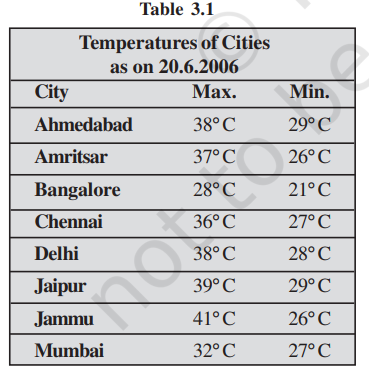3.2 Collecting Data:- We need to have data keeping in mind that specific information because all kinds of data will not be useful till we don’t know why we need them. So, for more specific learning, collecting data is essential and so has been briefly explained in this lesson.

3.3 Organisation Of Data:- Without proper organisation of data it won’t be readable and useful anymore. Therefore, the section 3.3 organisation of data has been given. This section covers how we can organise the data in tabular form as there are lots of data we come to access are represented in tabular format.

3.4 Representative Values:- In section 3.4 representative values the average has been discussed. Average lies between the highest and the lowest value of the given data so, we say average is a measure of the central tendency of the group of data. And so the knowledge of average is very useful as it helps us to represent the data and values.

3.5 Arithmetic Mean:- The most common representative value of the given group of data is Arithmetic Mean which can be calculated using the Mean formulas:-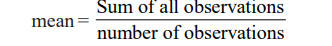To understand this term and its formulas consider some of the best examples mentioned in the NCERT Class 7th maths textbooks chapter 3 Data Handling. Within section 3.5, you will also find the subsection: 3.5.1 Range.

3.6 Mode:- The mode is another measure of central tendency or representative values. As per the requirements of data different representative values are used and mode is one of them.

As per the NCERT textbook, The mode of a set of observations is the observation that occurs most often.

Sometimes the data is so huge that we can’t handle it properly so another method is used, the Mode of Large Data. It is covered in 3.6.1 subsection.

3.7 Median:- In data handling, the core task is to find the measure of central tendency but Arithmetic mean and Mode are sometimes inappropriate methods so, Median is used. Median refers to the value which lies in the middle of the data (when values are arranged in an increasing or decreasing order).

There is an interesting small task given in NCERT Book to perform the above given methods. Try them: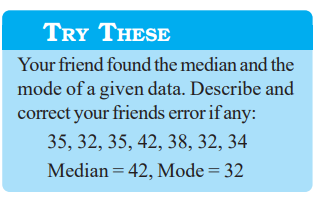3.8 Use Of Bar Graphs With A Different Purpose:- There is a great saying, A picture says thousand words. Thus, to grasp the data more accurately and fastly, we use bar graphs. When we collect the data and use the representative values, we try to represent them in graphs: Bar graphs, it helps us to get information real quick.

The bar graphs are affected by the scale we use hence, in 3.8.1 Choosing a Scale has been discussed.

3.9 Chance And Probability:- We use these terms in our daily life but, in mathematics it plays an important role. Since data handling is the term related to the list of data/numbers or events, we use chance and probability to understand the topic better. Go through the 3.9 Chance And Probability to understand its terms individually.

There are 24 questions in NCERT Class 7th Chapter 3 which helps students to practice plenty of questions.

Data Handling Chapter 3 Exercises

Ex. 3.1 – 9 Questions

Ex. 3.2 – 5 Questions

Ex. 3.3 – 6 Questions

Ex. 3.4 – 4 Questions

Study Tips for Class 7 Data Handling

Solve the given questions of graph, bar graph, double bar graphs, etc. as much as possible. Once you learn them, you can try to use the real world data to represent them. Doing this will enable you to take interest in these topics as well as it will clear your doubts.

#### Simple Equations (Chapter 4)

There are only 4 exercises in this chapter 4 that help students to learn how to simply convert a statement into a simple equation.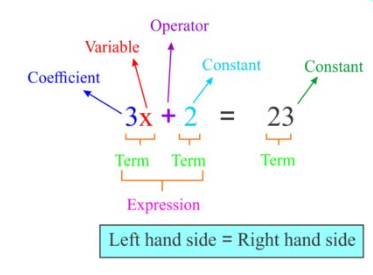As a result of learning this, students get an idea of how to intercept simple equations into real life.

4.1 A Mind-Reading Game!:- To allow students to understand the topic's simple equation, a section 4.1 A Mind-reading game is given. Although, it is not going to be a tough topic to grasp because you have already covered the algebra topic in class 6th and hopefully you have revised them before starting this lesson.

4.2 Setting Up Of An Equation:- Since majority of simple equation questions are in the format of word problem, the section 4.2 deals with setting up of an equation. So, it is recommended to all the students to go through the section 4.2 given in NCERT Class 7th Mathematics book. For the easy explanation, the book contains simple and real life examples.

4.3 Review Of What We Know:- Before diving deep into this topic, section 4.3 has covered some of the important points for the review purpose such as variables. It is a term that is used in Simple equations where it can change as per the requirements. Most importantly, Variables help in forming an equation.

4.4 What Equation Is?:- Equation contains Equity sign, apart from this there are more essential related terms (LHS and RHS) that need to be understood in order to form the equation. Hence, section 4.4 helps students know what equation is and its related terms.

Furthermore, under this section. It also explained how to solve equations in step by step methods.

4.5 More Equations:- To learn transposing a number section 4.5 contains more equations. It helps students to go through several examples to understand the equations more easily and precisely.

There is a term transposing a number, it is the core topic of this subsection. The transposing a number is used instead of adding or subtracting it from both sides of the equation. The below given image has some of the examples to understand this term more precisely: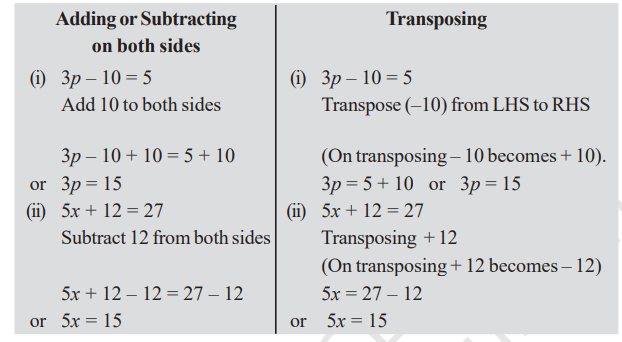4.6 From Solution To Equation:- By considering an interesting example, the book has tried to explain how someone can reverse the equations: From Solution to Equation.

There are some of the examples as well as some easy to solve questions given in the exercise.

4.7 Applications Of Simple Equations To Practical Situations:- The section 4.7 deals with some of the real world puzzle games. Since, it is important to understand why we are studying this topic otherwise we may lose the interest in study.

In order to answer the questions asked in this section, a student may have to use all the terms and methods they have studied earlier in this chapter.

Simple Equations Chapter 4 Exercises

Ex. 4.1 – 6 Questions

Ex. 4.2 – 4 Questions

Ex. 4.3 – 4 Questions

Ex. 4.4 – 4 Questions

Study Tips for Class 7 Simple Equations

Converting a statement to an equation, finding the value of an equation by addition and subtraction on both sides and solving the word problem is the main course of action for this chapter so, make sure you have developed a good comprehensive skill as it will help you to break down the statement into chunks, to convert them into simple equations.

18 questions with 6 long answer types and remaining all the questions are medium to easy.

Go through them one by one to learn the topics in detail.

#### Lines and Angles (Chapter 5)

The lines and angles are the basic geometry which has been introduced in NCERT 7th chapter 5.

Since we are surrounded by various forms of lines and angles, it is important to learn how to measure them.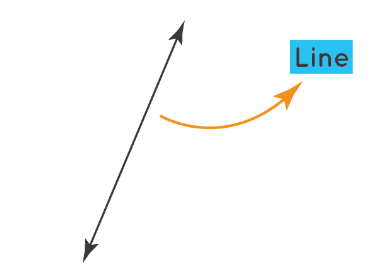The chapter 5 is a beginning of this topic where the properties of Lines, Angles, intersection, etc. are introduced.

5.1 Introduction:- Previously the students have been taught to identify different lines, line segments and angles in a given shape. However, here in this 5.1 introduction various shapes are given and students are told to identify different line segments and angles.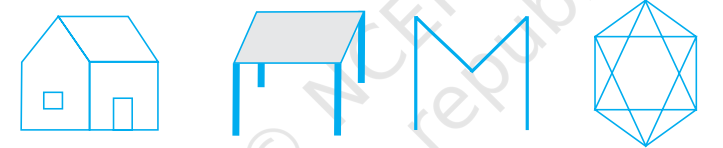Apart from this, there are various definitions given which help students to recall their past learnings of line, line segment, etc.

5.2 Related Angles:- There are various types of angles such as complementary angle, supplementary angles, Adjacent angle, Linear Pair, etc. All of these are very important so these have been covered in various sub sections such as:

• 5.2.1 Complementary Angles
• 5.2.2 Supplementary Angles
• 5.2.3. Adjacent Angles
• 5.2.4 Linear Pair
• 5.2.5 Vertically Opposite Angles

5.3 Pairs Of Lines:- Lines are an important part of chapter 5 and so the following pair of lines are given:

• 5.3.1 Intersecting Lines
• 5.3.2 Transversal
• 5.3.3. Angles made by a Transversal
• 5.3.4 Transversal of Parallel Lines

All students must have a thorough knowledge of this and to read about these they should go to the NCERT Class 5 Mathematics book.

5.4 Checking For Parallel Lines:- After gathering the knowledge of lines and angles the section 5.4 tries to help students to find When two lines are given, is there any proper method to check if they are parallel or not. The term perpendicular, transversal, corresponding angles, etc. have been discussed.

Based on this, there are some of the interesting questions given in the exercise.

A student must solve these questions to develop a deeper knowledge in this topic.

Lines and Angles Chapter 5 Exercises

Ex 5.1 - 14 Questions

Ex 5.2 - 6 Questions

Study Tips for Class 7 Lines and Angles

Geometry is an interesting topic however, in class 7th it covers the basics only so go through the properties of lines angles, intersection, complementary angles, supplementary angles, adjacent angles, linear pairs and solve some questions to understand them.

Apart from this, try to imagine the lines and angles as it will clear all the basics understanding of this topic.

#### The Triangle and Its Properties (Chapter 6)

This lesson covers some important topics: Drawing the median and altitude of triangles, determining the exterior angles, and finding the value of unknown angles, isosceles and equilateral triangles and more.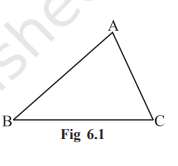6.1 Introduction:- This chapter starts with the definition of a triangle that is a closed figure of three line segments. Students are already aware of what line segments are. So in this section, they will be able to grasp in depth knowledge of triangles.

6.2 Medians Of A Triangle:- A median divides a triangle at a point on the line segment. For better understanding consider this: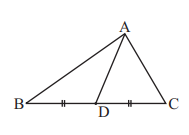Here in the above given image, the triangle ABC has three vertices A, B, & C. Now if we draw a line AD, it will divide the triangle into two. The point D where it divides the triangle is known as Medians of a triangle.

To understand this term more precisely refer to the NCERT Class 7th Maths book chapter 6 section 6.2 MEDIANS OF A TRIANGLE.

6.3 Altitudes Of A Triangle:- Section 6.3 has elaborated the altitudes of a triangle. As per the definition An altitude has one endpoint at a vertex of the triangle and the other on the line containing the opposite side. With the help of each vertex, an altitude can be drawn.

6.4 Exterior Angle Of A Triangle And Its Property:- The property of an exterior angle says, an exterior angle of a triangle is equal to the sum of its interior opposite angles.

To understand this condition or property refer to the Class 7th NCERT Maths book, as it helps you to know this thing in step-by-step method.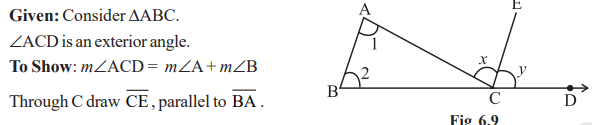The condition given in the above has been elaborated in the book more precisely.

6.5 Angle Sum Property Of A Triangle:- There is a very strong statement that says, The total measure of the three angles of a triangle is 180°. The Section 6.5 Angle Sum Property Of A Triangle has covered this statement fully and has explained it in small steps.

6.6 Two Special Triangles: Equilateral And Isosceles:- Equilateral triangles are called when all three sides are equal whilst Isosceles triangles are known as the triangle which has only two sides equal.

An example of Equilateral triangle:-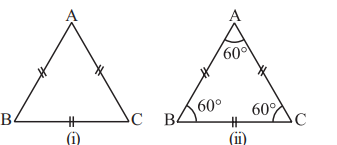An example of Isosceles triangle: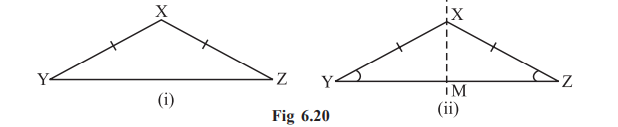These are special cases and so these two special triangles have been covered in this chapter.

6.7 Sum Of The Lengths Of Two Sides Of A Triangle:- Section 6.7 helps students to study the property of a triangle that is  the sum of the lengths of any two sides of a triangle is greater than the third side.

This statement has been proven in Chapter 6 of NCERT Class 7 Mathematics.

6.8 Right-Angled Triangles And Pythagoras Property:- This is an interesting property of a right angled triangle in which each side of a Right-Angled Triangle has its name.

For instance: The side opposite to the right angle is called the hypotenuse; the other two sides are known as the legs of the right-angled triangle.

The Pythagoras formula is as given: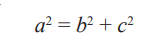Questions to practice based on these topics are available throughout the 5 exercises.

The Triangle and Its Properties Chapter 6 Exercises

Ex 6.1 - 3 Questions

Ex 6.2 - 2 Questions

Ex 6.3 - 2 Questions

Ex 6.4 - 6 Questions

Ex 6.5 - 8 Questions

Study Tips for Class 7 The Triangle and Its Properties

This lesson could be a bit tricky to study as well as a bit difficult to comprehend in the beginning. However, as you start practicing the questions of triangles and its properties, you will begin to find it easy.

Our advice for this chapter 6 is to pay attention to the properties of all the terms defined in each exercise of Triangle and Its Properties.

#### Congruence of triangles (Chapter 7)

This lesson discusses the most important topics of congruence: SSS, SAS, ASA, and RHS. Including these topics, this chapter also frames the base of congruence between any two figures and how to apply them effectively.The NCERT 7th maths chapter seven consists of only 2 exercises, where students will learn about these topics in a brief.

7.1 Introduction:- The topics that have been studied so far are enough for the introduction of Congruence. The Section 7.1 of NCERT Class 7 Maths Chapter 7 is ideal to start the topic of Congruence. In this section the definition of Congruence is given as well as where it implies. As per the introduction section the Congruence is ideal for three-dimensional shapes but, the focus of this chapter is on teaching the students about congruence in two dimensional shapes or plane figures.

7.2 Congruence Of Plane Figures:- Method of superposition has been discussed in section 7.2, so that a learner can easily understand the term congruence.

7.3 Congruence Among Line Segments:- By continuing the method of superposition, the Congruence Among Line Segments have been covered in section 7.3. An interesting conclusion of this section is given: If two line segments have the same or equal length, they are called congruent. Also, if two line segments are congruent, then they will have the same length.

7.4 Congruence Of Angles:- Having the same measure in two angles will be known as the Congruence Of Angles. As well as if two angles are congruent their measures will be the same.

7.5 Congruence Of Triangles:- After explaining about line segment congruence, simple shapes congruent and angle shape congruent the topics further extended to the Congruence Of Triangles. By applying the same method of superposition, the triangle congruence has been explained.
A few great and step by step examples have been given in the section 7.5

7.6 Criteria For Congruence Of Triangles:- We can’t use the method of superposition all the time so, there should be some solid method to verify the congruency of a triangle. Thus, in this section 7.6, the Criteria For Congruence Of Triangles have been discussed in the form of game for the clear understanding: A game, SSS game, SAS game, ASA game.

7.7 Congruence Among Right-Angled Triangles:- The above explained Criteria For Congruence Of Triangles doesn’t apply on right-angled triangles because in this triangle the angles are same for two similar triangles. Therefore, to give the special attention on Congruence Among Right-Angled Triangles, the section 7.7 has been explained.

Congruence of Triangles Chapter 7 Exercises

Ex 7.1 - 4 Questions

Ex 7.2 - 10 Questions

Study Tips for Class 7 Congruence of Triangles

No matter what chapter you study in 7th mathematics, if you want to understand the chapter and their terms, you have to relate them to real life.

So, after reading these topics, try to visualise the real life examples of Congruence of Triangles.

Another tip is to understand each term carefully and precisely, such as what is congruence? How many types of congruence are there? Etc. You need to be curious to develop an interest in mathematics.

#### Comparing Quantities (Chapter 8)

Converting fractional numbers and decimals to percentages, finding the percent increase or percent decrease, as well as profit and loss and annual interest questions are the parts of chapter 8 comparing quantities.

8.1 Introduction:- The chapter 8 of NCERT Class 7 maths helps students to get an idea of  what comparing quantities means and where it can be used. The terms of the ratio have been explained and a remark has been given that the ratio doesn’t have any units.

8.2 Equivalent Ratios:- If two like fractions are equal, we say the given ratios are equivalent. Sometimes we need to compare the equivalent ratios, so the method has been explained in section 8.2. In Class 6, the importance of equivalent ratios has been explained. So, the ratios which are equivalent are said to be in proportion and by the relevant examples this term has been recalled here in this section.

8.3 Percentage – Another Way Of Comparing Quantities:- In NCERT Class 7 Mathematics chapter 8 the term percentage has been introduced which is another way of comparing quantities. As per the NCERT, the Percentages are numerators of fractions with denominator 100. The definition of percentage as well as the origin of the percentage and converting a variety of numbers into the percentage are discussed. It has been explained in 5 sub sections: 8.3.1 Meaning of Percentage, 8.3.2 Converting Fractional Numbers to Percentage, 8.3.3 Converting Decimals to Percentage, 8.3.4 Converting Percentages to Fractions or Decimals, 8.3.5 Fun with Estimation.

8.4 Use Of Percentages:- After learning the relevant topics of percentage the section 8.4 contains four subsections, where the use of percentage has been elaborated such as in sub section 8.4.1 Interpreting Percentages, 8.4.2 Converting Percentages to “How Many”, 8.4.3 Ratios to Percents, 8.4.4 Increase or Decrease as Per Cent. With the help of section 8.4 students can learn how to use percentage in real life?

8.5 Prices Related To An Item Or Buying And Selling:- This portion of chapter 8 includes the topics such as cost price, selling price, Profit or Loss as a Percentage. Hence, this part is completely dedicated to the Prices Related To An Item Or Buying And Selling.

8.6 Charge Given On Borrowed Money Or Simple Interest:- Principal, Interest, simple interest are some of the most important topics to learn as it helps in real life finding the Charge Given On Borrowed Money Or Simple Interest. There are some important formulas in this portion that must be understood by all the students.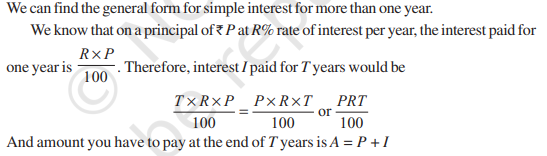There are a total of 24 questions having different difficulty levels. This chapter not only helps you to prepare for the exam but assists you to apply such learnings into real life.

Comparing Quantities Chapter 8 Exercises

Ex 8.1 - 3 Questions

Ex 8.2 - 10 Questions

Ex 8.3 - 11 Questions

Study Tips for Class 7 Comparing Quantities

The topics studied in this lesson are quite helpful for real life. Thus, the tip we will give you would be, to apply these learnings in your real life.

Apart from this, use the NCERT Solutions for class 7 maths to solve the complex questions in a step-by-step method.

#### Rational Numbers (Chapter 9)

In NCERT Chapter 9 of Class 7th, the rational numbers and their terms are introduced. Number line representation and comparison of rationals, as well as multiplying, dividing, adding, etc. have been briefly explained.

In this grade, this topic builds the foundation which will be used in higher standards.

9.1 Introduction:-In the intro part of chapter 9, it is mentioned what rational numbers are and what we shall learn here in this lesson. The number system has been extended here in this lesson. The concept of rational numbers along with their addition, subtraction, multiplication and division operations are the topics that are taught in this whole chapter.

9.2 Need For Rational Numbers:- Have you ever questioned why we need rational numbers? The section 9.2 helps you understand the need for Rational Numbers with some suitable examples.

9.3 What Are Rational Numbers?:- What is the origin of rational numbers and which numbers can be said as rational numbers are taught here in this section 9.3. Numerator and Denominator have been also covered here in this section.

9.4 Positive And Negative Rational Numbers:- The term positive rational number and Negative rational numbers have been defined in section 9.4. As per the definition, if there is a rational number whose numerator and denominator are positive, then it is called Positive Rational Numbers whilst if any one numerator or denominator will be negative then the number will be known as negative rational number.

9.5 Rational Numbers On A Number Line:- In this section the basic knowledge of showing integers on a number line has been extended by helping students understand how to represent the rational numbers on a number line.

9.6 Rational Numbers In Standard Form:- There is a standard form of Rational Numbers: When A rational number denominator is a positive integer and the numerator and denominator have no common factor other
than 1 is known as the standard form of rational number.

9.7 Comparison Of Rational Numbers:- The basic knowledge of comparing two integers is further extended in this section: how to compare the rational numbers. There are several relevant examples in this section that help students to better understand this term.

9.8 Rational Numbers Between Two Rational Number:- Note that, We can find an unlimited number of rational numbers between any two rational numbers. And the method of doing this has been explained in part 9.8 of chapter 9.

9.9 Operations On Rational Numbers:- You may have thought what operations on Rational Numbers can we do?, so the 9.9 section of chapter nine has explained this. There are some basic operations; such as Addition, Subtractios, Multiplications, & divisions, we have learned to do with integer numbers. So, here in this part of chapter the same operations: Addition, Subtractions, Multiplications, & Divisions On Rational Numbers have been taught.

There are two exercises and 14 questions that allow students to develop their fundamental knowledge.

Rational Numbers Chapter 9 Exercises

Ex 9.1 - 10 Questions

Ex 9.2 - 4 Questions

Study Tips for Class 7 Rational Numbers

The study tip for rational numbers is to recall the learning of LCM & HCF. Also, practice tons of questions to increase the calculation process.

#### Practical Geometry (Chapter 10)

This lesson has 5 exercises and each contains the questions on practical geometry, where students are taught how to use geometrical instruments.

There are plenty of questions in this chapter as well that examine the student’s basic learnings of SSS, SAS, ASA, and RHS congruence.

Along with this, students are taught how to use the geometrical instruments to construct transversals and parallel lines.

10.1 Introduction:- By giving a brief of some relevant shapes the introduction section helps students to know what they will learn in this chapter such as, how to draw parallel lines and some types of triangles.

10.2 Construction Of A Line Parallel To A Given Line, Through A Point Not On The Line:- The chapter begins by giving a very simple task: to construct the A Line Parallel To A Given Line, Through A Point Not On The Line. This question has been solved in 7 simple steps using ruler and compasses only.

10.3 Construction Of Triangles:- By using the knowledge of Congruence of Triangles and its properties, the construction of triangles has been taught in this portion of the chapter.

10.4 Constructing A Triangle When The Lengths Of Its Three Sides Are Known (SSS Criterion):- In this part, the book has taught students how to construct a triangle using ruler and compass when all of its three sides are given.

10.5 Constructing A Triangle When The Lengths Of Two Sides And The Measure Of The Angle Between Them Are Known. (SAS Criterion):- Similar to other constructions, here in this part it has been covered how to construct a triangle using the SAS criteria, meaning when measure of two sides and one angle is given. In Exercise 10.3 the questions are given on the same criteria.

10.6 Constructing A Triangle When The Measures Of Two Of Its Angles And The Length Of The Side Included Between Them Is Given. (ASA Criterion):- ASA or Angle-Side-Angle is a criteria that applies to a triangle so, this portion has covered the construction of such a triangle when two Angles are given and measure of one side are given. The section 10.6 contains a step by step method of this construction.

10.7 Constructing A Right-Angled Triangle When The Length Of One Leg And Its Hypotenuse Are Given (RHS Criterion):- Till now, you have learned a lot of methods to construct a triangle when their angle and sides measures are given. But, the Right-Angled Triangle is a special case in congruence and so a different approach is required to construct the Right-Angled Triangle. Here, in this action, students will be able to construct a right angled triangle When The Length Of One Leg And Its Hypotenuse Are Given.

There are a total of 16 questions distributed throughout the 5 exercises.

Practical Geometry Chapter 10 Exercises

Ex. 10.1 - 3 Questions

Ex. 10.2 - 4 Questions

Ex. 10.3 - 3 Questions

Ex. 10.4 - 3 Questions

Ex. 10.5 - 3 Questions

Study Tips for Class 7 Practical Geometry

Although practical geometry is quite an interesting chapter and easy to comprehend too, in the beginning you may face difficulty in using the instruments.

So, it is advised to go through the basics constructions first, use them to construct the transversals and parallel lines and then proceed further.

#### Perimeter and Area (Chapter 11)

Triangle, Circle, Square, rectangle, etc. are 2D shapes and often we need to measure the area of such shapes.

Therefore, the chapter 11 Perimeter and Area are included in class 7th mathematics.

11.1 Introduction:-There are several plane figures in mathematics and this lesson is dedicated to teach students how to find Perimeter and Area of squares and rectangles. However a brief intro of this topic has been already given in class 6 mathematics but, here in this class the topics have been further extended.

11.2 Squares And Rectangles:- By recalling the basic formulas of area and perimeter this section has begun, where one word problem has been given and it has been solved by using the formulae.

Some of the most important formulas recalled here in this sections are:-

• Perimeter of a regular polygon = number of sides × length of one side
• Perimeter of a square = 4 × side
• Perimeter of a rectangle = 2 × (l + b)
• Area of a rectangle = l × b, Area of a square = side × side

Various relevant examples have been given and solved in this section. Two subsections that are Triangles as Parts of Rectangles and Generalising for other Congruent Parts of Rectangles are mentioned here.

11.3 Area Of A Parallelogram:-Apart from the square and triangle there are other shapes such as Parallelogram. So, this section has covered how to find the area of a land which is in the Parallelogram shape.There is a solid formula for doing this:
Area of parallelogram = Area of rectangle = length × breadth = l × b

11.4 Area Of A Triangle:- What if we need to find the area of a triangle for some reason. How can we then calculate the area of a triangle? Here in this section  11.4, the method and formulas has been mentioned:

Area of each triangle = 1/2(Area of parallelogram) = 1/2(base × height) (Since area of a parallelogram = base × height) = 1/2 (b×h)× (or 1/2 bh , in short)

There is a remark in this section: all the congruent triangles are equal in area but the triangles having equal area need not to be congruent.

11.5 Circles:-  The section 11.5, helps students to learn how to find an area of shape which is circular. The subsections have been discussed for : 11.5.1 Circumference of a Circle, 11.5.2 Area of Circle.

11.6 Conversion Of Units:- Area of each shape has some particular units, so this part helps students to learn how to convert units while measuring areas to another unit.

11.7 Applications:- All mathematics terms have some uses and so the section 11.7 Application lets students explore the possibilities and uses of the above learnings in real life events.

This chapter includes the topics, formulas and step wise methods to help students to find the area and perimeter of squares, rectangles, triangles, parallelograms, and circles.

Perimeter and Area Chapter 11 Exercises

There are 44 questions available in 4 exercises of NCERT Class 7 Maths Chapter 11.

Ex. 11.1 - 8 Questions

Ex. 11.2 - 8 Questions

Ex. 11.3 - 17 Questions

Ex. 11.4 - 11 Questions

Perimeter and Area Chapter 11 Important Formulas

1. Perimeter of a square = 4 × side
2. Perimeter of a rectangle = 2 × (length + breadth)
3. Area of a square = side × side
4. Area of a rectangle = length × breadth
5. Area of a parallelogram = base × height
6. Area of a triangle = ½ × base × height
7. Circumference of a circle = 2πr
8. Area of a circle = πr2

Study Tips for Class 7 Perimeter and Area

There are plenty of word problems in this lesson so all students must have good comprehensive skills to identify the given data in the statement.

Apart from this, a thorough understanding of formulas and its constant revision is highly advised to the students.

#### Algebraic Expressions (Chapter 12)

The chapter 12 of NCERT Class 7 maths has 25 sums that allow students to learn what an expression means and what are the components that make up an algebraic expression.

There are various algebra formulas that are used in this lesson to find the value of algebraic expression as well as performing arithmetic operations on them.

12.1 Introduction:- By giving the brief of this chapter, the introduction part contains how algebraic expressions are formed, how they can be combined, how we can find their values and how they can be used. In other words, this lesson is fully dedicated to allow students to learn about Algebraic Expressions.

12.2 How Are Expressions Formed?:- To form an algebraic expression the term variable and constant are important to learn which has been covered in section 12.2. Later on in this part, it is mentioned how are expressions formed?

12.3 Terms Of An Expression:- This part helps students to understand what terms of an expression and
their factors are. Factors of a term and Coefficients are introduced as well as examples are given on these terms.

12.4 Like And Unlike Terms:- When terms have the same algebraic factors such as n 2xy – 3x + 5xy – 4,
Here the terms 2xy and 5xy are, that means they are like terms. When terms have different algebraic factors, they are unlike terms.

12.5 Monomials, Binomials, Trinomials And Polynomials:- An expression can have multiple terms and such terms can have variations. Henc, the word Monomials (Contains one term only), Binomials (Contains two terms only), Trinomials (Contains three terms only) And Polynomials (Contains multiple terms) have been introduced.

12.6 Addition And Subtraction Of Algebraic Expressions:- We can also add and subtract the algebraic expressions so, this thing has been covered in the section 12.6. Since, an algebraic expression has multiple conditions and terms their addition and subtractions are affected due to this that have been also elaborated in this part: Adding and subtracting like terms, Adding and subtracting general algebraic expressions.

12.7 Finding The Value Of An Expression:- The value of an expression depends upon the value of the variable that forms the expression. So, here in this section Finding The Value Of An Expression has been covered.

12.8 Using Algebraic Expressions – Formulas And Rules:- Various formulas and rules can be written in algebraic expression and the same have been covered here in the section 12.8. The topics and formulas that have been covered in this section are - Perimeter formulas, Area formulas, Rules for number patterns, Some more number patterns, Pattern in geometry.

Algebraic Expressions Chapter 12 Exercises

8 simple questions, 10 a bit tougher and 7 complex problems are there which are given across the 4 exercises.

Ex. 12.1 - 7 Questions

Ex. 12.2 - 6 Questions

Ex. 12.3 - 10 Questions

Ex. 12.4 - 2 Questions

Study Tips for Class 7 Algebraic Expressions

To solve the questions of Algebraic expressions, you will need to learn the basics of mathematics skills such as Subtractions, Additions, Multiplications, & Divisions.

Make sure you are good with numbers to calculate faster, if you’re not, you can become one by practice. Once you are good at this, try to solve algebraic expressions and keep revising all the formulas mentioned in the book.

Apart from this, you can use the NCERT Solutions for Class 7 Maths.

#### Exponents and Powers (Chapter 13)

The exponents and powers are one of the most important topics to study as it helps in dealing with the large numbers.

Here in chapter 13, there are 3 exercises and 17 questions that help students to practice various questions as well as learn how to express a number in the form of an exponent.

Variety of exponentiation laws have been elaborated in this chapter too.

13.1 Introduction:- The section 13.1 helps students to know why we use exponents and powers. Actually to compare, read and to understand the large number, the exponents and variables are used. So, in this chapter, the exponents and powers as well as how to use them are explained.

13.2 Exponents:- What are the exponents? So, the exponents are the method of writing large numbers into short for example:- 10, 000 = 10 × 10 × 10 × 10 = 104

13.3 Laws Of Exponents:- There are some sorts of laws for exponents that are - Multiplying Powers with the Same Base, Dividing Powers with the Same Base, Taking Power of a Power, Multiplying Powers with the Same Exponents, Dividing Powers with the Same Exponents, Numbers with exponent zero. This section contains these topics briefly.

13.4 Miscellaneous Examples Using The Laws Of Exponents:- Contains some examples using rules of exponents so that students can develop a deeper and better grasp on the topic.

13.5 Decimal Number System:- Students will learn in this part on how to expand and represent a number into a decimal number system.

13.6 Expressing Large Numbers In The Standard Form:- If a decimal number is expressed as the product of a number between 1 and 10 and the integral power of 10 then it is a standard form. This is explicitly explained in section 13.6.

Exponents and Powers Chapter 13 Exercises

3 exercises and 17 questions ideally provide a good amount of problems to solve.

Ex. 13.1 - 8 Questions

Ex. 13.2 - 5 Questions

Ex. 13.3 - 4 Questions

Study Tips for Class 7 Exponents and Powers

There are 7 challenging questions and remaining all problems are easy to medium difficulty level. So, it is advised to the students to first develop a strong grip on the basic level questions.

Practice basic level exponents and powers questions as much as possible before moving to the difficult one.

#### Symmetry (Chapter 14)

Symmetry is an important part of design and the objects we see every day. Thus, this lesson will be very interesting because it covers the symmetry in detail.

Also, there are certain topics: line of symmetry & rotational symmetry that have been explained in this lesson thoroughly.

14.1 Introduction: Symmetry:- This portion contains a short intro of symmetry and where it is being used. Also, what we will learn in this chapter.

14.2 Lines Of Symmetry For Regular Polygons:- A shape has line symmetry when one half of it is the mirror image of the other half and so the regular polygons are symmetrical figures. With some relevant examples this topic has been covered here in this part: 14.2

14.3 Rotational Symmetry:- When an object is rotated at its own axis then all the time the shape of the object looks the same, thus it is known as Rotational Symmetry. The NCERT Class 7th Chapter 14 has elaborated this in quite a detail by referring to the real life examples.

14.4 Line Symmetry And Rotational Symmetry:- Some shapes have line symmetry whilst some have rotational symmetry but, there are also some conditions in which a shape has both: Line Symmetry And Rotational Symmetry. This is what this section has covered in detail with real life examples.

There are 19 sums in this lesson which are important to solve and practice in order to score good marks in the examination.

Symmetry Chapter 14 Exercises

Line symmetry, Regular polygons, Centre of Rotation, Angle of rotation, Order of rotational symmetry have been elaborated explicitly in these 3 exercises.

Ex. 14.1 - 10 Questions

Ex. 14.2 - 2 Questions

Ex. 14.3 - 7 Questions

#### Visualising Solid Shapes (Chapter 15)

Chapter 15 Visualising Solid Shapes is an important chapter as it builds the foundation of mensuration questions. This lesson helps students to identify the different elements of a solid figure.

15.1 Introduction: Plane Figures And Solid Shapes:- This chapter is ideal to learn or identify the plane figures and solid shapes. Mainly this lesson covers the foundation of 3 dimensional shapes that we are surrounded with such as balls, ice-cream cones etc.

15.2 Faces, Edges, and Vertices:- The Faces, Edges, and Vertices are already introduced to the students but, in this section, these topics are elaborated in detail for solid shapes such as cube and more. Cube has 8 corners which are called its vertices, 12 line segments of a cube form its edges and 6 flat square surfaces are known as its faces.

15.3 Nets For Building 3-D Shapes:- A net is a kind of skeleton-outline in 2-D (two dimensional) that forms a 3D shape when it is being folded on its edges. The section 15.3 covers it in detail by performing a real world task.

15.4 Drawing Solids On A Flat Surface:- Oblique sketch and Isometric Sketches have been discussed in this section. Both of these are methods or techniques to draw 3D shapes on a flat 2D surface by distorting the image somewhat. Doing this act is known as visual illusion. Both of these techniques are elaborated in detail by taking a real life example.

15.5 Viewing Different Sections Of A Solid:- We can see a 3-D Shape in various ways or methods. One Way to View an Object is by Cutting or Slicing, Second way is by shadow play, the third way of viewing a shape is by Looking at the shape from Certain Angles. There are very interesting relevant examples and subtopics are given in section 15.5.

4 exercises and 15 questions are ideal to learn this chapter thoroughly. There are several techniques discussed in this chapter which help students to view different sections of a solid.

Visualising Solid Shapes Chapter 15 Exercises

In this lesson, 9 are very simple, 3 are slightly difficult while 2 are complex sums.

Ex. 15.1 - 5 Questions

Ex. 15.2 - 5 Questions

Ex. 15.3 - 1 Questions

Ex. 15.4 - 3 Questions

### CBSE Class 7 Mathematics Unit-wise Marks Weightage

The below given table includes the unit wise marking for CBSE Class 7 Mathematics.

 Sr No Topic/Unit Marks 1 Integer 15% 2 Fractions and Decimals 17.5% 3 Data Handling 17.5% 4 Simple Equations 17.5% 5 Lines and Angles 17.5% 6 Triangle and its Properties 12% 7 Congruence of a Triangle 12% 8 Comparing Quantities 14% 9 Rational Numbers 7% 10 Practical Geometry 12% 11 Perimeter and Area 14% 12 Algebraic Expressions 13% 13 Exponents and Powers 14% 14 Symmetry 10% 15 Visualising Solid Shapes 5%

### Benefits of Using Class 7 Maths NCERT SolutionsSince these NCERT Maths Solutions are prepared by maths experts, it is very obvious to experience some of the exceptional benefits.

1. Maths questions and its answers could be confusing but the step by step approach in the solutions book enables students to solve questions in a comprehensive manner.
2. Each question may require a different set of formulas so by accessing the Class 7 Maths NCERT Solutions, students can easily take help of the formulas as it is given between each step.
3. The solutions not only help in solving the complex questions, but also allow students to deepen their basic knowledge.

### Importance of Class 7 Maths SolutionsThe class 7 Maths Solutions can play an important part in a student’s learnings by helping them in Exam Preparation, Clearing doubts, and boosting the time management skills.

Here are the brief about the importance of 7th standard maths solutions:

1. In Exam Preparation:- During the exam preparation students are already running out of time. That's why they aren’t able to pay attention to each question one by one. However, it is important to go through each question of 7th maths to score better. With the help of NCERT Class 7 Maths Solutions this task seems possible as the solutions contain step by step answers of each problem in detail.
2. In Clearing Doubts to Boost Confidence :- Doubts lower the confidence level and in maths having doubts is very normal. Therefore Solutions help students a lot in clearing doubts in 7th mathematics by explaining each question briefly.
3. In Learning How To Manage Time

The Learning process is not straight forward, there are various ups and downs and imbalance in time management skills a student has to face. But the NCERT 7th Maths solutions enables students to learn how to manage time by providing the solutions of all easier to complex questions. Due to this, students get an opportunity to finish their maths syllabus within the set time frame.

#### FAQs, on NCERT Solutions for Class 7 MathsFAQs
Need answers? Find them here...

Every single topic given in class 7 NCERT Maths are interlinked with each other. Therefore, you should definitely practice all Questions Provided in NCERT Solutions Class 7 Maths. If you face any problems while solving them, you can refer to the NCERT Class 7 Chapter wise Solutions. It is free.

Since NCERT Solutions for Class 7 Maths consists of step by step answers to all the questions as per the latest syllabus to make the topics easier to understand. So, it becomes important for students to have NCERT Solutions for Class 7 Maths.

In order to download NCERT Chapter Wise Solutions for Class 7 Free, students can visit Selfstudys website. Their solutions are prepared by the subject matter experts after analysing the difficulty level of questions. It can ease the process of solving the questions of mathematics.

Majority of students find Chapter 13 – Exponents and Powers a bit tough. You may find other or no chapters tough.

There are 15 chapters in class 7 Maths that cover the topics from Integers to Visualising Solid Shapes.

The higher you can score the better percentage you will get in class 7. However, in general 60% marks is considered as the first division and more than 80% is known as outstanding performance.

You can easily learn maths in 7th class by paying attention to every individual chapter and topic in detail. You can firstly refer to the Class 7 Maths Syllabus to understand what topics you have to cover and then with the help of Maths textbooks you can go through each of them one by one to learn.

The syllabus of NCERT 7 Maths is a bit lengthy, there are a total of 15 chapters to cover. To know the syllabus in detail download them from Selfstudys.

Getting full marks could be easier for you, if you follow a proper time table, practice questions more often, and revise the studied topics time and again. After doing all these things, you can do a self-assessment to check how well you have prepared to get full marks. If you score full in your self-assessment test,more likely you will score full marks in 7th class.

Related News
See All×
Open Now